# ValueAtG

Model ElementValueAtG computes the value of a signal,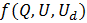when a second signal,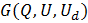, achieves a certain value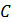.

ValueAtG

## Description

ValueAtG is defined as follows:(1)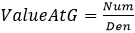Where: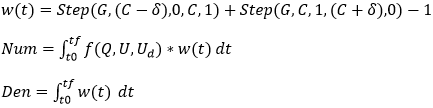## Attribute Summary

Name Property Modifiable by Command? Designable
label Str ()
fsignal Function ()   Yes
gsignal Function ()   Yes
gvalue Double ()
delta Double ()
scale Double (1)

## Usage

# ValueAtG used to compute fsignal at a particular value of gsignal
ValueAtG (fsignal=fsignal, gsignal=gsignal, gvalue=gvalue, delta=delta, optional_attributes)

## Attributes

Defined as value of a function f when another function g reaches a particular value.
fsignal
String
A MotionSolve expression defining the function for which ValueAtG is required.

fsignal attribute is mandatory.

gsignal
String
A MotionSolve expression defining the function at which fsignal is required.
gsignal is mandatory.
gvalue
Double
Value of gsignal at which fsignal is to be computed.
gvalue is mandatory.
delta
Double
Interval around the gvalue to be used in the step function for the calculation of fsignal.
delta is mandatory.
Optional attribute
label
String
Specifies the name of the ValueAtG object.
This attribute is optional. When not specified, MotionSolve creates a label for you.
scale
Double
Specifies the scale factor to scale the ValueAtG value. If not specified, it defaults to 1.0.
This attribute is optional.

## Example:

# Compute the steer angle difference when the steering wheel angle is ZERO

# Steering wheel angle
swa = "AZ(i,j)".format(i=m77.id,j=m66.id)

# Steering angle difference
sad = "YAW(i,j) - YAW(k,l)".format(i=m33.id, j=m22.id, k=m55.id, l=m44.id)

# Steer angle difference at zero SWA
result = ValueAtG (
label = "Steer Angle Difference at Zero SWA",
fsignal = swa,
)